Using the transform matrix on canvas

Until now, we have been dealing with transformations that are intuitive and does not need a matrix-level understanding of the transformation. However, in order to use the Transformation() method, it is important to have a basic understanding of matrix manipulation. So here we go.

Matrix Manipulation

If you are working with 2-D, you can basically represent any point on the canvas in the matrix form. For example, if you have a point (x,y), it can be represented as: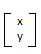Suppose we want to translate/move this point (x,y) to another location say (x1,y1), this change in location can be achieved by adding dx to the x coordinate and dy to the y coordinate where dx = x1-x and dy = y1-y. So we will essentially be doing a matrix addition as follows: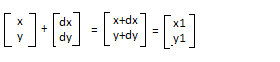While points represented as 2 X 1 matrices work for simple transformations, complex transformations may require that they be represented as homogeneous coordinates. What this means is that instead of representing points as 2 X 1 matrices, we will be representing them as 3 X 1 matrices as follows.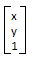In this case, the translation matrix would be written as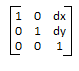and multiplied with the current transformation matrix to get the change of location instead of performing matrix addition.

So, the result after translation would be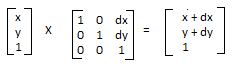The transform() method

The transform() method allows you to apply any transformation to the current matrix. So you can use it to do scaling, rotating, translation as well as many other complex transformations. The method takes six parameters:

transform(a, b, c, d, e, f)

where a,b,c,d,e, and f form the following transformation matrix: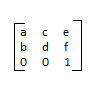The current transformation matrix gets replaced by the product of the current transformation matrix and the matrix provided in the transform() method.

 HTML Code     Translating an image on canvas
Try Code